# Neural Network From Scratch in Python

0
746## IntroductionTo Neural Network From Scratch in Python

In this article understand about the 3-layer neural network from scratch in Python. We explain the mathematical term as much as possible.

Don’t be confused in machine learning and deep learning concept, in this post I am assuming that you’re comfortable with the basic concept of machine learning, statistics, and calculus. Ideally, you required knowledge about optimization techniques like gradient descent algorithm.

Why from scratch?

Because it helps you gain an understanding of how neural network works, clear the mathematics term how it update the weight, bias, and many more terms.

Even if you have a Neural Network libraries like PyBrain. Once you did at least one time  is an extremely valuable exercise.

That’s why we are going to discuss Neural Network From Scratch in Python with coding examples.

## Machine Learning Algorithm

### Logistics Regression Algorithm

To demonstrate the point let’s train a Logistic Regression classifier. Its input will be the x- and y-values and the output of the predicted class (0 or 1). Because this algorithm works on binary classification, that’s why predict class 0 or 1. To make our life easy we use the Logistic Regression class from scikit-learn.

See the result the graph shows the decision boundary by logistic regression classifier. It separates the data as good as it can using a straight line, but it’s unable to capture the “moon shape” of our data. It means can not separate perfectly by the edge to edge.

So, let’s train the Neural Network.

Widget not in any sidebars

## Deep Learning

### Training the Neural Network

A neural network is a supervised learning algorithm which means that we provide it the input data containing the independent variables and the output data that contains the dependent variable.

In the beginning, the neural network makes some random predictions, these predictions are matched with the correct output and the error or the difference between the predicted values and the actual values is calculated. The function that finds the difference between the actual value and the propagated values is called the cost function. The cost here refers to the error. Our objective is to minimize the cost function. Training a neural network basically refers to minimizing the cost function.

Let’s now build a 3-layer neural network means one input layer(left side), one output layer(right side of the network), and in between one layer known as ‘Hidden Layer’. The number of nodes in the input layer is determined by the dimensionality of our data, 2. Similarly, the number of nodes in the output layer is determined by the number of classes we have, also 2. (Because we only have 2 classes we could actually get away with only one output node predicting 0 or 1, but having 2 makes it easier to extend the network to more classes later on). The input to the network will be x- and y- coordinates and its output will be two probabilities, for class 0 (“female”) and for class 1 (“male”). It looks something like this:

We can choose the dimensionality (the number of nodes) of the hidden layer. The more nodes we put into the hidden layer the more complex functions we will be able to fit. But higher dimensionality comes at a cost. Why cost? Because First, more computation is required to make predictions and learn the network parameters. A bigger number of parameters also means we become more prone to overfitting our data.

How to choose the size of the hidden layer? While there are some general guidelines and recommendations, it always depends on your specific problem and is more of an art than a science. We will play with the number of nodes in the hidden later on and see how it affects our output.

We also need to pick an activation function for our hidden layer. The activation function transforms the inputs of the layer into its outputs when the value matches i.e. after updating weight value. A nonlinear activation function is what allows us to fit nonlinear hypotheses. Common choices for activation functions are ReLU, sigmoid function, and tanh. We will use tanh, which performs quite well in many scenarios. A nice property of these functions is that their derivate can be computed using the original function value. For example, the derivative of is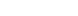. This is useful because it allows us to compute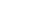once and re-use its value later on to get the derivative.

Because we want our network to output probabilities the activation function for the output layer will be the softmax, which is simply a way to convert raw scores to probabilities. If you’re familiar with the logistic function you can think of softmax as its generalization to multiple classes.

## Feed Forward

In the feed-forward part of a neural network, predictions are made based on the values in the input nodes and the weights. The weights of a neural network are basically the strings that we have to adjust in order to be able to correctly predict our output.

Step 1: (Calculate the dot product between inputs and weights)

The nodes in the input layer are connected with the output layer via three weight parameters. In the output layer, the values in the input nodes are multiplied with their corresponding weights and are added together. Finally, the bias term is added to the sum. The b in the above figure refers to the bias term.

The bias term is very important here. Suppose if we have a person who doesn’t smoke, is not obese, and doesn’t exercise, the sum of the products of input nodes and weights will be zero. In that case, the output will always be zero no matter how much we train the algorithms. Therefore, in order to be able to make predictions, even if we do not have any non-zero information about the person, we need a bias term. The bias term is necessary to make a robust neural network.

Mathematically, in step 1, we perform the following calculation:

X.W=x1w1+x2w2+x3w3+b

Widget not in any sidebars

Step 2: (Pass the result from step 1 through an activation function)

The result from Step 1 can be a set of values. However, in our output, we have the values in the form of 1 and 0. We want our output to be in the same format. To do so we need an activation function, which squashes input values between 1 and 0. One such activation function is the sigmoid function.

The sigmoid function returns 0.5 when the input is 0. It returns a value close to 1 if the input is above 0.5. In the case of negative input, the sigmoid function outputs a value close to zero.

Mathematically, the sigmoid function can be represented as:

θX.W=11+e−X.W

#put sigmoid curve

the input is positive the output is close to 1. However, the output is always between 0 and 1. This is what we want.

This sums up the feedforward part of our neural network. It is pretty straightforward. First, we have to find the dot product of the input feature matrix with the weight matrix. Next, pass the result from the output through an activation function, which in this case is the sigmoid function. The result of the activation function is basically the predicted output for the input features.

## Back Propagation

The principle behind the working of a neural network is simple. We start by letting the network make random predictions about the output. We then compare the predicted output of the neural network with the actual output. Next, we fine-tune our weights and the bias in such a manner that our predicted output becomes closer to the actual output, which is basically known as “training the neural network”.

In the backpropagation section, we train our algorithm. Let’s take a look at the steps involved in the backpropagation section.

Step 1: (Calculating the cost)

The first step in the backpropagation section is to find the “cost function” of the predictions. The cost of the prediction can simply be calculated by finding the difference between the predicted output and the actual output. The higher the difference, the higher the cost will be.

Step 2: (Minimizing the cost)

Our ultimate purpose is to fine-tune the knobs of our neural network in such a way that the cost is minimized. If your look at our neural network, you’ll notice that we can only control the weights and the bias. We cannot control the inputs, we cannot control the dot products, and we cannot manipulate the sigmoid function.

In order to minimize the cost, we need to find the weight and bias values for which the cost function returns the smallest value possible. The smaller the cost, the more correct our predictions are.

This is an optimization function where we have to find the function minima. To find the minima of a function, we can use the gradient descent algorithm.

Basically find the partial derivative of the cost function with respect to each weight and bias and subtract the result from the existing weight values to get the new weight values.

The derivative of a function gives us its slope at any given point. To find if the cost increases or decreases, given the weight value, we can find the derivative of the function at that particular weight value. If the cost increases with the increase in weight, the derivative will return a positive value which will then be subtracted from the existing value.

On the other hand, if the cost is decreasing with an increase in weight, a negative value will be returned, which will be added to the existing weight value since negative into negative is positive.

How our Network makes Prediction

Our network makes predictions using forward propagation, which is just a bunch of matrix multiplications and the application of the activation function(s) we defined above. If x is the 2-dimensional input to our network then we calculate our prediction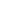(also two-dimensional) as follows: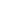is the input of layerand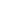is the output of layerafter applying the activation function.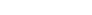are parameters of our network, which we need to learn from our training data. You can think of them as matrices transforming data between layers of the network. Looking at the matrix multiplications above we can figure out the dimensionality of these matrices. If we use 500 nodes for our hidden layer then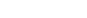,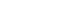,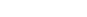,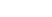. Now you see why we have more parameters if we increase the size of the hidden layer.

Generating Dataset

Let’s start by generating a dataset with use of a scikit-learn library. We will go with the make_moons function.

Now, the result shows have two classes, plotted as red and blue points. You can think of the blue dots as male patients and the red dots as female patients, with the x- and the y-axis being medical measurements.

Our aim is to train a Machine Learning classifier that predicts the correct class (male or female) given the x- and y- coordinates.

NOTE: The data is not linearly separable, we can’t draw a straight line that separates the two classes. This means that linear classifiers, such as Logistic Regression, won’t be able to fit the data unless you hand-engineer non-linear features (such as polynomials) that work well for the given dataset.

In fact, that’s one of the major advantages of Neural Networks. The hidden layer of a neural network will learn features for you.

Import required library

import numpy as np

import sklearn

#from sklearn import datasets

from sklearn import *

from matplotlib import pyplot as plt

# Generate a dataset and plot it

np.random.seed(0)

X, y = sklearn.datazasets.make_moons(200, noise=0.20)

plt.scatter(X[:,0], X[:,1], s=40, c=y, cmap=plt.cm.Spectral)

def plot_decision_boundary(pred_func):
# Set min and max values and give it some padding
x_min, x_max = X[:, 0].min() - .5, X[:, 0].max() + .5
y_min, y_max = X[:, 1].min() - .5, X[:, 1].max() + .5
h = 0.01
# Generate a grid of points with distance h between them
xx, yy = np.meshgrid(np.arange(x_min, x_max, h), np.arange(y_min, y_max, h))
# Predict the function value for the whole gid
Z = pred_func(np.c_[xx.ravel(), yy.ravel()])
Z = Z.reshape(xx.shape)
# Plot the contour and training examples
plt.contourf(xx, yy, Z, cmap=plt.cm.Spectral)
plt.scatter(X[:, 0], X[:, 1], c=y, cmap=plt.cm.Spectral)
# Train the logistic rgeression classifier
clf = sklearn.linear_model.LogisticRegressionCV()
clf.fit(X, y)

# Plot the decision boundary
plot_decision_boundary(lambda x: clf.predict(x))
plt.title('Logistic Regression')Implement the Neural Network

Draw a sigmoid curve
input = np.linspace(-10, 10, 100)

def sigmoid(x):
return 1/(1+np.exp(-x))

from matplotlib import pyplot as plt
plt.plot(input, sigmoid(input), c="r")We start by defining some useful variables and parameters for gradient descent:

num_examples = len(X) # training set size
nn_input_dim = 2 # input layer dimensionality
nn_output_dim = 2 # output layer dimensionality

# Gradient descent parameters (I picked these by hand)
epsilon = 0.01 # learning rate for gradient descent
reg_lambda = 0.01 # regularization strength

First, let’s implement the loss function.
# Helper function to evaluate the total loss on the dataset
def calculate_loss(model):
W1, b1, W2, b2 = model['W1'], model['b1'], model['W2'], model['b2']
# Forward propagation to calculate our predictions
z1 = X.dot(W1) + b1
a1 = np.tanh(z1)
z2 = a1.dot(W2) + b2
exp_scores = np.exp(z2)
probs = exp_scores / np.sum(exp_scores, axis=1, keepdims=True)
# Calculating the loss
corect_logprobs = -np.log(probs[range(num_examples), y])
data_loss = np.sum(corect_logprobs)
# Add regulatization term to loss (optional)
data_loss += reg_lambda/2 * (np.sum(np.square(W1)) + np.sum(np.square(W2)))
return 1./num_examples * data_loss
# Helper function to predict an output (0 or 1)
def predict(model, x):
W1, b1, W2, b2 = model['W1'], model['b1'], model['W2'], model['b2']
# Forward propagation
z1 = x.dot(W1) + b1
a1 = np.tanh(z1)
z2 = a1.dot(W2) + b2
exp_scores = np.exp(z2)
probs = exp_scores / np.sum(exp_scores, axis=1, keepdims=True)
return np.argmax(probs, axis=1)
# This function learns parameters for the neural network and returns the model.
# - nn_hdim: Number of nodes in the hidden layer
# - num_passes: Number of passes through the training data for gradient descent
# - print_loss: If True, print the loss every 1000 iterations
def build_model(nn_hdim, num_passes=20000, print_loss=False):

# Initialize the parameters to random values. We need to learn these.
np.random.seed(0)
W1 = np.random.randn(nn_input_dim, nn_hdim) / np.sqrt(nn_input_dim)
b1 = np.zeros((1, nn_hdim))
W2 = np.random.randn(nn_hdim, nn_output_dim) / np.sqrt(nn_hdim)
b2 = np.zeros((1, nn_output_dim))

# This is what we return at the end
model = {}

# Gradient descent. For each batch...
for i in xrange(0, num_passes):

# Forward propagation
z1 = X.dot(W1) + b1
a1 = np.tanh(z1)
z2 = a1.dot(W2) + b2
exp_scores = np.exp(z2)
probs = exp_scores / np.sum(exp_scores, axis=1, keepdims=True)

# Backpropagation
delta3 = probs
delta3[range(num_examples), y] -= 1
dW2 = (a1.T).dot(delta3)
db2 = np.sum(delta3, axis=0, keepdims=True)
delta2 = delta3.dot(W2.T) * (1 - np.power(a1, 2))
dW1 = np.dot(X.T, delta2)
db1 = np.sum(delta2, axis=0)

# Add regularization terms (b1 and b2 don't have regularization terms)
dW2 += reg_lambda * W2
dW1 += reg_lambda * W1

W1 += -epsilon * dW1
b1 += -epsilon * db1
W2 += -epsilon * dW2
b2 += -epsilon * db2

# Assign new parameters to the model
model = { 'W1': W1, 'b1': b1, 'W2': W2, 'b2': b2}

# Optionally print the loss.
# This is expensive because it uses the whole dataset, so we don't want to do it too often.
if print_loss and i % 1000 == 0:
print('quot;Loss after iteration %i: %f" %(i, calculate_loss(model))')

return model

# Build a model with a 3-dimensional hidden layer
model = build_model(3, print_loss=True)

# Plot the decision boundary
plot_decision_boundary(lambda x: predict(model, x))
plt.title('quot;Decision Boundary for hidden layer size 3"')


## Conclusion

In this article, We discussed about Neural Network From Scratch in Python. we created a very simple neural network with one input, one output layer, and a hidden layer from scratch in Python. Linearly separable data is the type of data that can be separated by a hyperplane in n-dimensional space.

Real-word artificial neural networks are much more complex, powerful, and consist of multiple hidden layers and multiple nodes in the hidden layer. Such neural networks are able to identify non-linear real decision boundaries.